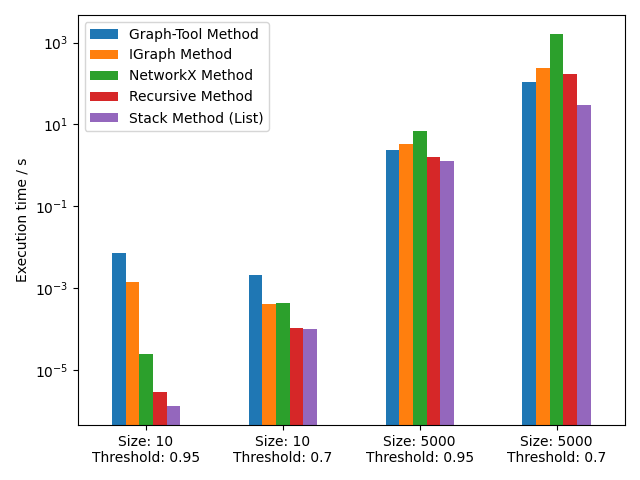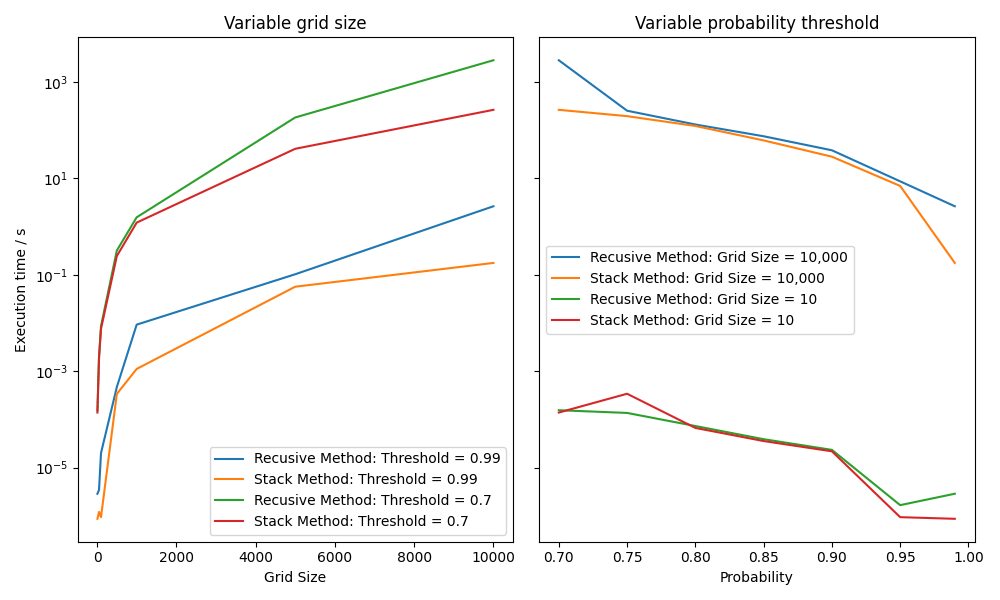## Introduction

This final entry in the series will attempt to summarize the results presented so far in terms of the different solutions found, and explore the different parameters in the problem that might influence the appropriate solution.

I’ve written 5 different solutions to this problem, in order of implementation they are:

• A recursive depth-first search
• Using NetworkX
• Using iGraph
• Using graph-tools
• A stack-based depth-first search

To figure out which approach is the most efficient, I need a DoE that takes into consideration the different parameters that might affect performance. I’ve already done some investigations into the amount of time taken building the network compared to finding the clusters in the network, and some approaches are likely more suited to situations where the average reservoir size is higher/lower.

To investigate this, the first thing I looked into was this dependence of the average reservoir size on the grid size and probability. It’s not strictly necessary to understand which approach is most performant, but I thought it might be an interesting thing to investigate.

## Number of reservoirs vs probability and grid size

This isn’t really a property of the solution, it’s more of a property of the problem itself. Here’s a plot of how the average reservoir size (and therefore the ratio of active sites to grid size) changes as a function of the Probability Threshold (i.e. the number between 0 and 1 I use to determine if a site is ‘active’ or not) and the grid size. I’m only giving one dimension, but the grid itself is square.Figure 1: Dependence of grid size and probability threshold on average reservoir size

If there were no clustering I’d expect this to be dependent on probability threshold only, although with much higher variability at low grid size because the sample size is so much smaller. However, the clustering step adds a dependency on grid size for small grid sizes only. This is because I’m not using any boundary conditions in my grid, it’s just a hard edge. For a grid size of 10×10, there are 100 elements, but 32 of those only have 5 neighbors instead of 8. And 4 only have 3 neighbors! The table below summarizes the average number of neighbors per site for each grid size:

There’s a pretty clear correlation here between the drop off of average neighbors and the drop off of average reservoir size. Once the grid gets to 100×100 then the average number of neighbors is within 98% of 8 neighbors in an infinite grid, so we’d expect 100×100 to be the first grid with a static average reservoir size, and that’s basically what figure 1 shows.

I’m not a mathematician, but I’ve got a pretty strong feeling that there’s an analytical relationship between the grid size and the number of neighbors. There’s nothing here that really relates to the puzzle, but it’s an interesting geometrical quirk of the way I’m building the grid. Getting to the bottom of this is something else to add to the backlog!

## Designing the Experiment

Anyway, back to the problem at hand. It’s clear that both the Probability Threshold and Grid Size have an impact on the problem, so my DoE was to run at grid sizes of 10-10000 in logarithmic increments, and probability thresholds of 0.99 (obviously 1.00 is trivial) and 0.95 to 0.6 in 0.05 increments. I was previously unable to hit 10,000×10,000 due to RAM constraints, but I recently treated myself to another 16 gig, so it’s now within reach!

To avoid letting this run for ages, I started off just running the 4 extreme cases with the entire set of algorithms. The idea being if I see 2 implementations that are fastest on all 4 cases, I’ll run the entire DoE on those 2 only.

But disaster! I hit a recursion limit with the threshold set to 0.6! Even by allowing a higher recursion limit with s`ys.setrecursionlimit` Python was still segfaulting, which means I was probably hitting my system stack size limit. I could have increased this stack size, but I decided just to back the DoE limit off to 0.7 instead. I did check the populated fraction of the grid at a threshold of 0.6, and it had climbed to an impressive 63%! The default Python recursion limit is 1000, so I must have had a reservoir that exceeded that limit.

Here are the results of my sparse run for all solutions at those four extremes of the parameter ranges (yes, I stole this plot from the previous article):Figure 2: Comparison of execution times for all methods for extreme parameter values

It’s clear here that the Stack and Recursive methods are the fastest, so I’ll disregard the external library solutions for the rest of the analysis.

## Recursive Vs Stack

So let’s take a deeper look into it. Here’s the data for the full range of solutions for both the recursive and the stack solutions.Figure 3: Performance of recursive vs stack DFS algorithms

I experimented with a surface plot to show the explicit dependence on both parameters with all the cross-terms included, but it was difficult to really see what was going on. So I settled instead on the slices along the edges of the parameter space.

The stack approach performs either similar to or better than the recursive approach in all situations, so it’s clearly the optimum solution. However, there are some situations, both at small grids and for medium probabilities, where the difference between them really isn’t that great. I’m not really sure why this is, but it must be that the time saved in not doing a whole load of recursing balances against the set subtractions required in the stack approach.

Set subtraction is an O(n) operation, so the total time performing a set subtraction scales linearly with the grid size. The recursion however becomes more of an issue as the average reservoir size increases. To see how this is the case, if the average reservoir size was 1, then no recursion would be necessary at all. Therefore, large reservoir sizes will have a disproportionately negative effect on the recursive approach compared to the stack approach. So with all that being said, I don’t know why I’m seeing the behavior I am. Figure 3 shows that the difference between the two seems more dependent on the grid size than the probability threshold, and figure 1 shows that reservoir size is dependent on the probability threshold only beyond a grid size of 100.

The only reason I can think of is that there’s some other effect that’s slowing the recursive method down more for increasing grid size which is dwarfing the real effect of recursive vs stack. One possible culprit might be the set copy operation I perform at the beginning of the recursion, so I can continue to find neighbors of the site in question. It would make sense that as the set gets very large it becomes more expensive to copy it.

If this were the other way around and I was seeing a performance hit in the stack implementation I would look into it more. However, i know that the stack implementation is the right one in Python, so I’m going to leave it as is. However, another task for the backlog is to see if I can get rid of that set copy operation somehow and try to improve performance of the recursive approach.

## List vs Deque

The final step is to investigate which stack is the most performant for this particular scenario. I mentioned previously that both lists and deques can be used for this, since they both support FILO accessing. Here’s the results of running the same set of experiments on a list version of the code and a deque version of the code.Figure 4: Effect of list vs deque on the performance of the stack algorithm

There’s really no difference here. With the exception of the results at 5000, 0.99 an 10, 0.75, they behave pretty much identically. This isn’t surprising, since the average size of the stack is probably around 20 items since at most we have a large forest of very small trees.

## Conclusions and Discussion

So, the best implementation here is the stack-based implementation using the deque-based stack, which is pretty uncontroversial. However, is this actually a practical solution if this were a real-world problem? To start thinking about this, I’m going to focus on the North Sea.

There doesn’t seem to be any information easily available on the total sizes that are surveyed, but the North Sea itself is 220,000 square miles, so that’s a fair place to start. The maximum grid size that I considered here was 10,000×10,000, which when mapped to the North Sea would give a resolution of approximately 2.2 * 10-3 square miles (1.5 acres, 0.5 hectares). That’s probably way too small a grid size, a size of 500×500 would give a resolution of 0.88 square miles, which seems much more reasonable.

There are 173 active rigs in the North Sea. My crude model for building and clustering the grid with a probability threshold of 0.95 generated a 500×500 grid with about 300 reservoirs, so it’s not a million miles away from reality. If this were required to be production code then obviously I’d be given the grid to work with, however the signs here point to Python being a perfectly performant solution for this scenario.

However, there are two realistic-type extensions that I can immediately think of that might be required here.

The first is that we are treating this scenario as a 2-dimensional investigation only. The mathenatics of this solution could be easily translated into 3 or more dimensions, but obviously the 3-dimensional case is the only extenson that’s actually physically useful. Oil deposits typically form in the strata of the continental shelf, so this would probably only require the addition of 10 or so sites in the z direction.

The second is that there may be other analytics required beyond just determining the number and size of the reservoir. Some simple additions could be made to the code, but if any other analytics were needed that more closely followed a graph-theory type analysis, I’d certainly move to implement a library instead of writing custom code. It would then depend on the trade-off between maintainability vs performance vs licensing requirements as to which library I’d go for:

• graph-tool is the most performan, but it’s LGPL licensed and is a huge pain to compile
• NetworkX is the slowest, but it’s a simple pip install, even on Windows, and it’s released under a BSD license, so there’s no issues with commercial reuse

## Wrap-Up

So that ends this investigation! It was a simple throw-away question in an interview, but the question had some really interesting mathematical features and potential solutions, so I thought it was worth digging into in a bit more detail.

I plan to polish and publish the code, and then I’ll start working on my next project. I have a few ideas, but I’m happy for any inspiration on what I can do next!